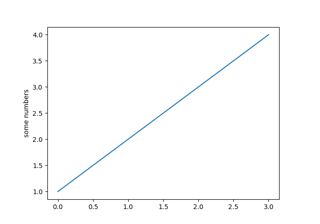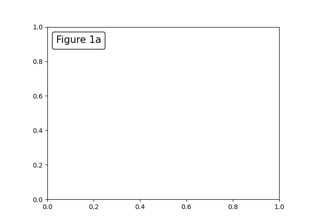# matplotlib.pyplot.annotate#

matplotlib.pyplot.annotate(text, xy, *args, **kwargs)[source]#

Annotate the point xy with text text.

In the simplest form, the text is placed at xy.

Optionally, the text can be displayed in another position xytext. An arrow pointing from the text to the annotated point xy can then be added by defining arrowprops.

Parameters
textstr

The text of the annotation.

xy(float, float)

The point (x, y) to annotate. The coordinate system is determined by xycoords.

xytext(float, float), default: xy

The position (x, y) to place the text at. The coordinate system is determined by textcoords.

xycoordsstr or `Artist` or `Transform` or callable or (float, float), default: 'data'

The coordinate system that xy is given in. The following types of values are supported:

• One of the following strings:

Value

Description

'figure points'

Points from the lower left of the figure

'figure pixels'

Pixels from the lower left of the figure

'figure fraction'

Fraction of figure from lower left

'subfigure points'

Points from the lower left of the subfigure

'subfigure pixels'

Pixels from the lower left of the subfigure

'subfigure fraction'

Fraction of subfigure from lower left

'axes points'

Points from lower left corner of axes

'axes pixels'

Pixels from lower left corner of axes

'axes fraction'

Fraction of axes from lower left

'data'

Use the coordinate system of the object being annotated (default)

'polar'

(theta, r) if not native 'data' coordinates

Note that 'subfigure pixels' and 'figure pixels' are the same for the parent figure, so users who want code that is usable in a subfigure can use 'subfigure pixels'.

• An `Artist`: xy is interpreted as a fraction of the artist's `Bbox`. E.g. (0, 0) would be the lower left corner of the bounding box and (0.5, 1) would be the center top of the bounding box.

• A `Transform` to transform xy to screen coordinates.

• A function with one of the following signatures:

```def transform(renderer) -> Bbox
def transform(renderer) -> Transform
```

where renderer is a `RendererBase` subclass.

The result of the function is interpreted like the `Artist` and `Transform` cases above.

• A tuple (xcoords, ycoords) specifying separate coordinate systems for x and y. xcoords and ycoords must each be of one of the above described types.

See Advanced Annotations for more details.

textcoordsstr or `Artist` or `Transform` or callable or (float, float), default: value of xycoords

The coordinate system that xytext is given in.

All xycoords values are valid as well as the following strings:

Value

Description

'offset points'

Offset (in points) from the xy value

'offset pixels'

Offset (in pixels) from the xy value

arrowpropsdict, optional

The properties used to draw a `FancyArrowPatch` arrow between the positions xy and xytext. Defaults to None, i.e. no arrow is drawn.

For historical reasons there are two different ways to specify arrows, "simple" and "fancy":

Simple arrow:

If arrowprops does not contain the key 'arrowstyle' the allowed keys are:

Key

Description

width

The width of the arrow in points

The width of the base of the arrow head in points

The length of the arrow head in points

shrink

Fraction of total length to shrink from both ends

?

The arrow is attached to the edge of the text box, the exact position (corners or centers) depending on where it's pointing to.

Fancy arrow:

This is used if 'arrowstyle' is provided in the arrowprops.

Valid keys are the following `FancyArrowPatch` parameters:

Key

Description

arrowstyle

the arrow style

connectionstyle

the connection style

relpos

see below; default is (0.5, 0.5)

patchA

default is bounding box of the text

patchB

default is None

shrinkA

default is 2 points

shrinkB

default is 2 points

mutation_scale

default is text size (in points)

mutation_aspect

default is 1.

?

any key for `matplotlib.patches.PathPatch`

The exact starting point position of the arrow is defined by relpos. It's a tuple of relative coordinates of the text box, where (0, 0) is the lower left corner and (1, 1) is the upper right corner. Values <0 and >1 are supported and specify points outside the text box. By default (0.5, 0.5) the starting point is centered in the text box.

annotation_clipbool or None, default: None

Whether to draw the annotation when the annotation point xy is outside the axes area.

• If True, the annotation will only be drawn when xy is within the axes.

• If False, the annotation will always be drawn.

• If None, the annotation will only be drawn when xy is within the axes and xycoords is 'data'.

**kwargs

Additional kwargs are passed to `Text`.

Returns
`Annotation`

## Examples using `matplotlib.pyplot.annotate`#Pyplot tutorial

Pyplot tutorialAnnotations

Annotations